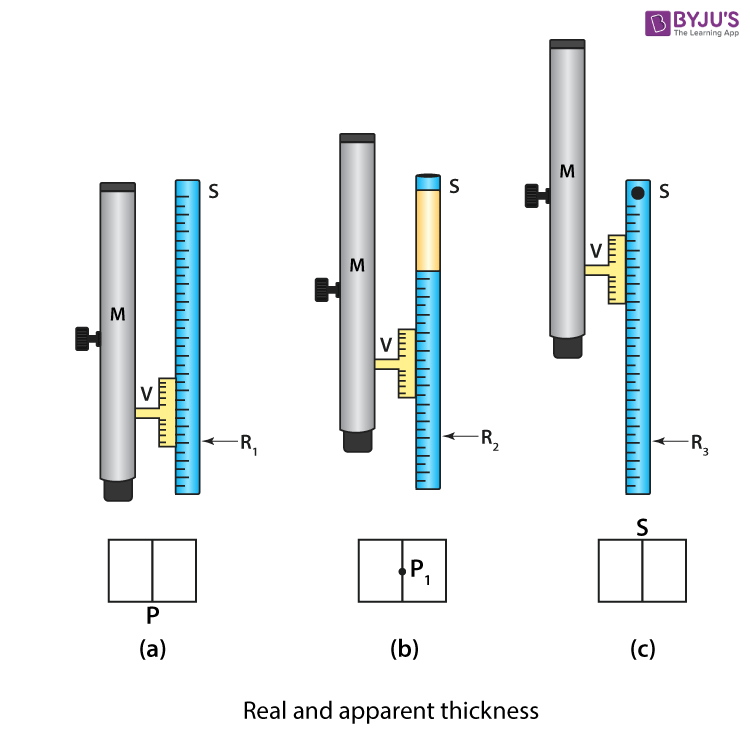# To Determine Refractive Index of a Glass slab using a Travelling Microscope

A Travelling microscope is a compound microscope that is fitted on a vertical scale. It carries a vernier scale along the main scale and can be moved upward or downward. Below is an experiment to determine refractive index of a glass slab using a travelling microscope.

## Aim

To determine the refractive index of a glass slab using a travelling microscope.

## Materials Required

1. 3 glass slabs of different thicknesses but the same material
2. A travelling microscope
3. Lycopodium powder

## Theory

The principle behind glass slab

When a glass slab is placed on a horizontal surface, and its bottom surface is viewed from the top, it appears to be elevated due to refraction. The apparent thickness of the slab is determined by the distance between the apparent bottom and the top of the glass slab. The refractive index with respect to the medium and air is given as:

$$\begin{array}{l}n=\frac{real\,thickness\,of\,the\,slab}{apparent\,thickness\,of\,the\,slab}\end{array}$$

## Diagram## Procedure

Adjustment of a travelling microscope

1. To get sufficient light, place the travelling microscope (M) near the window.
2. To make the base of the microscope horizontal, adjust the levelling screw.
3. For clear visibility of the cross wire, adjust the position of the eyepiece.
4. For the vertical scale of the microscope, determine the vernier constant.
5. Mark point P on the microscope’s base using black ink.
6. To avoid the parallax between the cross-wires and the mark P, make the microscope vertical and focus on P.
7. Let R1 be the vernier scale and main scale reading on the vertical scale.
8. Place the glass slab with the least thickness over the mark P.
9. Let P1 be the image of the cross mark. Move the microscope upwards and focus on P1.
10. For reading, R2 on the vertical scale repeat step 7.
11. Sprinkle a few particles of lycopodium powder on the slab’s surface.
12. To focus the particle near S, raise the microscope further upward.
13. For reading, R3 on the verticle scale repeat step 7.
14. Repeat the above steps for different thickness glass slabs.
15. Record the observations.

## Observations And Calculations

Vernier constant for the vertical scale of microscope = ……..cm

Table for microscope readings

 Sl.no Reading on a vertical scale when a microscope is focused on Real thickness (R3 – R1) in cm Apparent thickness (R3 – R2) in cm Refractive index $$\begin{array}{l}n=\frac{R_{3}-R_{1}}{R_{3}-R_{2}}\end{array}$$ Cross mark without slab R1 in cm Cross mark with slab R2 in cm Lycopodium powder R3 in cm 1. 2. 3.

Mean,

$$\begin{array}{l}n=\frac{n_{1}+n_{2}+n_{3}}{3}\end{array}$$

## Result

The ratio

$$\begin{array}{l}\frac{R_{3}-R_{1}}{R_{3}-R_{2}}\end{array}$$
is constant and gives the refractive index of the glass slab.

## Precautions

1. The parallax in a microscope should be removed properly.
2. To avoid backlash error, the microscope should be moved upward.

## Sources Of Error

1. The scale used in the microscope might not be calibrated properly.
2. The lycopodium powder layer on the glass slab might be thick.

## Viva Questions

Q1. Define normal shift.

Ans: Normal shift is defined as the difference between actual depth and apparent depth.

Q2. What causes a normal shift?

Ans: Normal shift is caused due to the refraction of light.

Q3. What is the SI unit of normal shift?

Ans: The SI unit of normal shift is a metre.

Q4. What is apparent shift?

Ans: Apparent shift is defined as the difference between the object’s distance from the refracting surface and the image distance from the refracting surface.

Q5. On what factors does the apparent depth depend?

Ans: Following are the factors on which the apparent depth depend:

1. Nature of the medium
2. Thickness of medium
3. Colour of light

Stay tuned with BYJU’S to learn more about other Physics-related experiments.

Test Your Knowledge On To Determine Refractive Index Of A Glass Slab Using A Travelling Microscope!